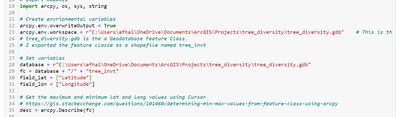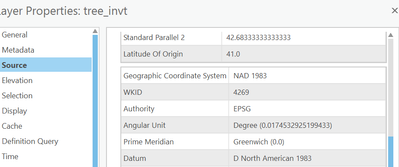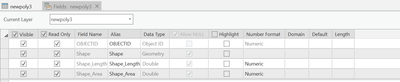# CreateFeatureclass Display Issue

736
6
11-19-2020 02:55 PM
Labels (1)New Contributor II

[Edited to correct mistype] I am doing a bit of coding with Python. Here is the relevant bit of unremarkable code to create a polygon object.

sr = 4269

polygon = arcpy.Polygon(array,sr)
bound_box = "newpoly3"
arcpy.management.CreateFeatureclass(database,bound_box,"POLYGON","","","",sr)

with arcpy.da.InsertCursor(bound_box,"SHAPE@") as cursor:
cursor.insertRow([polygon])

This code reliably works when I specify the spatial reference in a projected coordinate system, e.g. 2249. However, when I set the spatial reference in a geographic coordinate system, e.g. 4269, there's an issue: the shape doesn't display. I went to Table of Contents --> newpoly3.shp --> Design --> Fields and checked the "Shape" box, but the shape still doesn't show up on my map. It's clearly been created; the attribute table shows an area and length. It just doesn't display. Anyone know what's up with it?

Here is the entire bit of code. The goal is to play with Python a bit and to form polygon from the x-y extent of a shapefile of points.Tags (1)
1 Solution

Accepted SolutionsbyMVP Esteemed Contributor

You need to project your points if there are originally in one coordinate system and you want to see them in another... see this simple example

`````` import arcpy
# Spatial reference set to GCS_WGS_1984
spatial_reference = arcpy.SpatialReference(4326)
pnt = arcpy.Point(-75.5, 45.5)
pnt_geometry = arcpy.PointGeometry(pnt, spatial_reference)
# change spatial reference
SR = "NAD 1983 CSRS MTM  9"
pnt_prj = pnt_geometry.projectAs(SR)

pnt_geometry
<Point (-75.5, 45.5, #, #)>

pnt_prj
<Point (382950.18492149055, 5040495.111721899, #, #)>``````

... sort of retired...
Tags (3)
6 RepliesbyMVP Esteemed Contributor

from your examples... one is string, the other number.... which one worked?

... sort of retired...New Contributor II

Oh, shoot. I pasted the wrong code there. Numbers worked; I never ran it with a string.byMVP Esteemed Contributor

You need to project your points if there are originally in one coordinate system and you want to see them in another... see this simple example

`````` import arcpy
# Spatial reference set to GCS_WGS_1984
spatial_reference = arcpy.SpatialReference(4326)
pnt = arcpy.Point(-75.5, 45.5)
pnt_geometry = arcpy.PointGeometry(pnt, spatial_reference)
# change spatial reference
SR = "NAD 1983 CSRS MTM  9"
pnt_prj = pnt_geometry.projectAs(SR)

pnt_geometry
<Point (-75.5, 45.5, #, #)>

pnt_prj
<Point (382950.18492149055, 5040495.111721899, #, #)>``````

... sort of retired...
Tags (3)New Contributor II

I think I'm not explaining clearly. Visual illustration:

The original layer file has two described coordinate systems. The projected one is 2249.The geographic coordinate system is 4269."newpoly1.shp" uses the projected coordinate system 2249 and shows up on the map. Here is the Zoom to layer of "newpoly1.shp"."newpoly3.shp" uses the geographic coordinate system 4269. (Same code, only difference is the number). Below is the "Zoom to layer" for "newpoly3.shp". As you can see (or not see), there is no shape.The shape field is checked to be visible.What I want to know... is why newpoly3.shp isn't showing up. Is it a matter of reprojection? I don't quite understand why, if so.byMVP Esteemed Contributor

The original layer file has two described coordinate systems.

You have a layer... tree_invt ... it can only have one coordinate system.
It is either projected (which it appears to be) or unprojected (it can't be both).
Simply "defining" the coordinate system as something else, just makes it wrong.
You have to "project" it using that tool to actually physically change the coordinates.
That is what my example shows, how you project from one coordinate system to another.
In order to do that, it has to be "defined" correctly in the first place

... sort of retired...New Contributor II

Hi Dan, I'm still a bit lost, but I appreciate the explanation and code--it does work when I specify a projection!